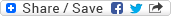## Sunday, June 1, 2008

### Concept of short-circuit operators in C or Java

Question: What's the concept of short-circuit operators in C or Java?

Answer: && and are the two short-circuit operators in C as well as in Java. Here short-circuit mean that if the outcome of the expression can be determined by the left operand only then the right operand won't be evaluated and hence the order of evaluation will always be from left to right irrespective of whether you place the operand within parentheses or not. For example:- suppose we have an expression as 'operand1 && operand2' and 'operand1' is evaluated to be 'false', then there is no point evaluating the right operand 'operand2' as irrespective of whether that operand is 'true' or 'false' the expression will always be 'false' only. Short-circuit operators have been given this extra feature just to save the time of useless computation in the cases where the result is already determined. If the 'operand1' returns 'true' then the result of the expression will depend upon what 'operand2' returns and hence in that case 'operand2' will also be evaluated. Similar explanation can be given for operator as well.

This left to right order of evaluation is guaranteed for one more operator - the comma operator, but that operator is not a short-circuit operator and hence all the operands will be evaluated.